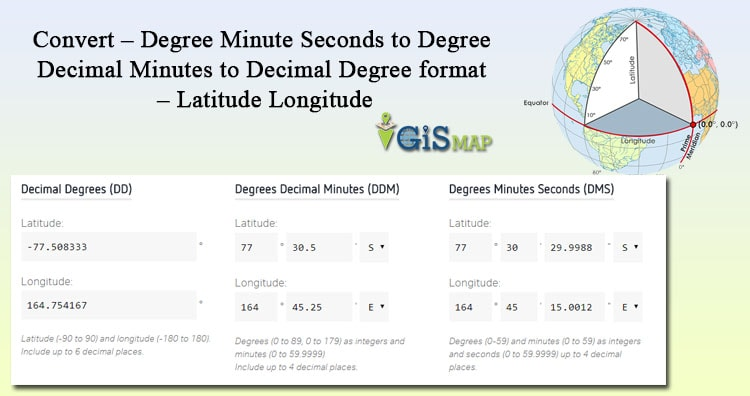# Convert – Degree Minute Seconds to Degree Decimal Minutes to Decimal Degree format – Latitude LongitudeConvert – Degree Minute Seconds to Degree Decimal Minutes to Decimal Degree format. Geographical location information is represented in terms of Latitude and Longitude, where Latitude gives the angular distance of a point from equator to elevation either north or south, and Longitude gives the angular distance of a point east or west of the meridian. This Angular distance values of Lat and Lon is either represented in Degree, Minute, Seconds(dd,mm,ss) number system or Decimal Degree number system. So let us discuss about, how to represent the location with Latitude and Longitude, their value range and how to convert them with the tool.

#### Latitude values Range:

Point on Equator line is calculated as 0° latitude, while North pole as 90°N and South pole as 90°S. Latitude values range in between 0° and 90° in positive
(representing north) and in negative (representing south) values.

#### Longitude values Range:

Prime Meridian or Greenwich meridian is defined as 0° longitude. Longitude values ranges from 0° to 180° east or from 0° to 180° west.

### Latitude and Longitude Representation – Different converted formats:

Generally in three formats, latitude and longitude values are represented. Let us take example of each format, by taking latitude longitude of San Antonio.
1.) Degree Minutes Seconds :
For example: 29° 25′ 26.5152″, -98° 29′ 37.6974″

2.) Degree Decimal Minutes:
For example: 29° 25.441916666667’ , -98° 29.6283’

3.) Decimal Degree:
For example: 29.424032, -98.493805

## Convert – Degree Minute Seconds to Degree Decimal Minutes to Decimal Degree format

Earth equator line is divided in 360 equal parts, each part represents a degree distance, where 1°=111.32 Km. Every Degree is further divided in 60 minutes. This means, 1 minute = 111.32/60 = 1.85 Km, and every minute is divided in 60 seconds. So 1 seconds = 1.85/60 = 0.0309 Km.

### Convert Degree Minute Seconds to Degree Decimal for the Latitude Longitude values:

As 1°=60’=3600”, so we can compute degree decimal by:

d = degree + minute / 60 + seconds/3600

Let us take an example: Compute 29° 25′ 26.5152″, -98° 29′ 37.6974″ to degree decimal:

Latitude to Degree Decimal = d = 29 + 25/60 + 26.5152/3600 = 29.42432
Longitude to Degree Decimal = d = -(98 + 29/60 + 37.6974/3600) = -98.493805

#### Tool to convert lat lon values from degree minute seconds to degree decimal:

LATITUDE :Degree= Minute= Seconds=

LONGITUDE :Degree= Minute= Seconds=

Latitude :

Longitude :

### Convert Degree Minute Seconds to Degree Decimal:

Again, as 1°=60’=3600”, we would compute Degree, Minute and Seconds separately from Degree Decimal.

• Degree = integer part of Decimal degree = int(ddd.dd) = ddd.
• Minute = integer part of ((Decimal degree – Degree computed above) * 60) = int(ddd.dd – ddd) * 60.
• Seconds = (Degree Decimal – degree – Minute/60) * 3600 = (ddd.dd – ddd – m/60)*3600

Let us take an example. Compute 29.424032, -98.493805 to Degree Minute Seconds format:

Degree = int(29.424032) = 29
Minute = int((29.424032 – 29) * 60) = int (25.44192) = 25
Seconds = (29.424032 – 29 – 25/60) * 3600 = 26.5152″

So latitude = 29° 25′ 26.5152”

Similarly computing longitude of -98.493805, we get the degree minute second to -98° 29′ 37.6974.

LATITUDE :
LONGITUDE :

Latitude :

Longitude :

### Convert – Degree Minute Seconds to Degree Decimal Minutes:

Fetch the Seconds number, and divide it by 60, and then add the result with minute,

For example: Convert 29° 25′ 26.5152” to Degree Decimal Minute:

• Here Seconds = 26.5152,
• so dividing it by 60 i.e 26.5152/60 = 0.44.
• Now add this with Minute, we will get 29° 25.44, which is in Degree Decimal Minutes format. If you multiply again 0.44 by 60 you will get again Degree Minute seconds format.

#### You may find further link, to find the tool for conversion of Latitude longitude format, with Map view:

Latitude Longitude format Conversion – Degree Decimal, Degree Minute Seconds and Degree Decimal Minutes

Do comment below, if you have any doubt or if you want share any other related information with us by commenting below.